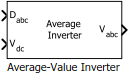# Average-Value Inverter

Compute three-phase AC voltage from inverter DC voltage

Since R2020a

•Libraries:
Motor Control Blockset / Electrical Systems / Inverters

## Description

The Average-Value Inverter block models an average-value and full-wave inverter. It computes the three-phase AC voltage output from inverter DC voltage by using the duty cycle information.

### Equations

These equations describe how the block computes the three-phase AC voltage.

`${D}_{0}=\frac{\left({D}_{a}+{D}_{b}+{D}_{c}\right)}{3}$`
`${V}_{a}={V}_{dc}×\left({D}_{a}-{D}_{0}\right)$`
`${V}_{b}={V}_{dc}×\left({D}_{b}-{D}_{0}\right)$`
`${V}_{c}={V}_{dc}×\left({D}_{c}-{D}_{0}\right)$`

where:

• ${D}_{a}$, ${D}_{b}$, and ${D}_{c}$ are the modulation indices ranging between 0 and 1.

• ${V}_{dc}$ is the DC bus voltage of the inverter (Volts).

• ${V}_{a}$, ${V}_{b}$, and ${V}_{c}$ are the output three-phase voltages (Volts).

## Ports

### Input

expand all

Three-phase modulation indices in the range [0,1] for generating voltages that run the motor.

Data Types: `single` | `double` | `fixed point`

DC bus voltage input to the inverter.

Data Types: `single` | `double` | `fixed point` | `uint8` | `uint16` | `uint32`

### Output

expand all

Three-phase voltage (Volts) corresponding to the input duty cycle that runs the motor.

Data Types: `single` | `double` | `fixed point`

## Version History

Introduced in R2020a